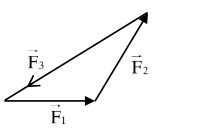# Statement :1 If three forces`
Question:

Statement :1

If three forces $\overrightarrow{\mathrm{F}}_{1}, \overrightarrow{\mathrm{F}}_{2}$ and $\overrightarrow{\mathrm{F}}_{3}$ are represented by three sides of a triangle and $\overrightarrow{\mathrm{F}}_{1}+\overrightarrow{\mathrm{F}}_{2}=-\overrightarrow{\mathrm{F}}_{3}$, then these three forces are concurrent forces and satisfy the condition for equilibrium.

Statement : II

A triangle made up of three forces $\overrightarrow{\mathrm{F}}_{1}, \overrightarrow{\mathrm{F}}_{2}$ and $\overrightarrow{\mathrm{F}}_{3}$ as its sides taken in the same order, satisfy the condition for translatory equilibrium.

In the light of the above statements, choose the most appropriate answer from the options given below:

1. Statement-I is false but Statement-II is true

2. Statement-I is true but Statement-II is false

3. Both Statement-I and Statement-II are false

4. Both Statement-I and Statement-II are true.

Correct Option: , 4

Solution:Here $\overrightarrow{\mathrm{F}}_{1}+\overrightarrow{\mathrm{F}}_{2}+\overrightarrow{\mathrm{F}}_{3}=0$

$\overrightarrow{\mathrm{F}}_{1}+\overrightarrow{\mathrm{F}}_{2}=-\overrightarrow{\mathrm{F}}_{3}$

Since $\overrightarrow{\mathrm{F}}_{\text {net }}=0$ (equilibrium)

Both statements correct